# Understanding Boxplots | Codementor

The image above is a boxplot. A boxplot is a standardized way of displaying the distribution of data based on a five number summary (“minimum”, first quartile (Q1), median, third quartile (Q3), and “maximum”). It can tell you about your outliers and what their values are. It can also tell you if your data is symmetrical, how tightly your data is grouped, and if and how your data is skewed.
This tutorial will include:

• What is a boxplot?
• Understanding the anatomy of a boxplot by comparing a boxplot against the probability density function for a normal distribution.
• How do you make and interpret boxplots using Python?

As always, the code used to make the graphs is available on my github. With that, let’s get started!

For some distributions/datasets, you will find that you need more information than the measures of central tendency (median, mean, and mode) as the image below shows (image taken from here)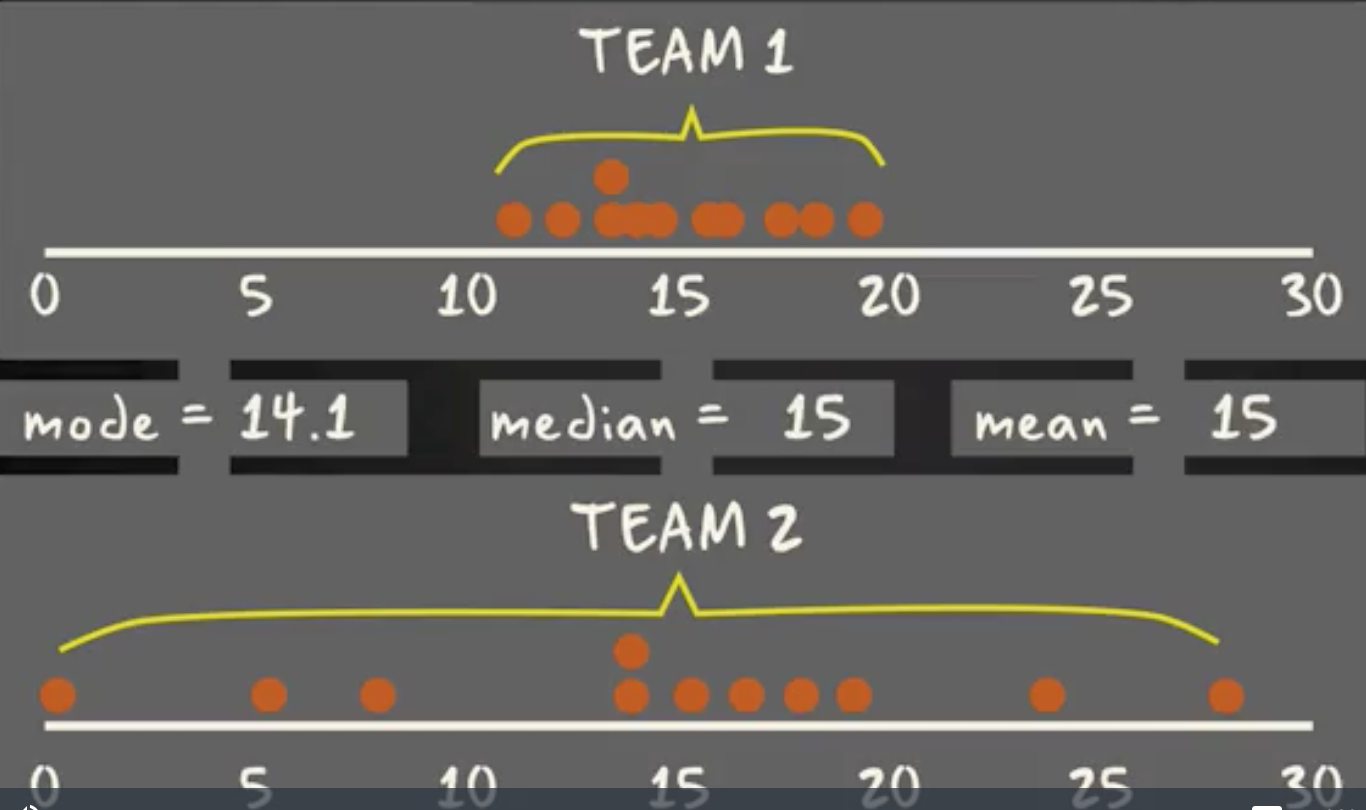You need to have information on the variability or dispersion of the data. A boxplot is a graph that gives you a good indication of how the values in the data are spread out. Although boxplots may seem primitive in comparison to a histogram or density plot, they have the advantage of taking up less space, which is useful when comparing distributions between many groups or datasets.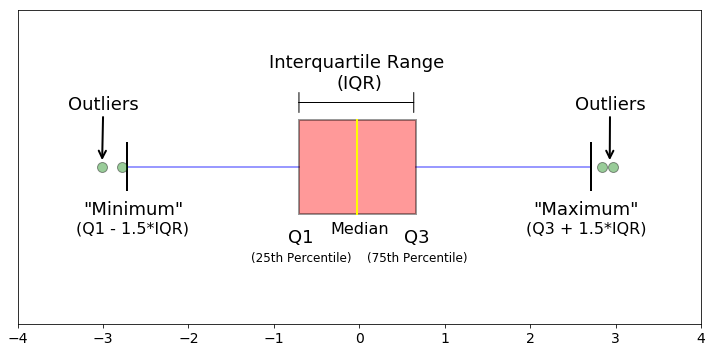Boxplots are a standardized way of displaying the distribution of data based on a five number summary (“minimum”, first quartile (Q1), median, third quartile (Q3), and “maximum”).

median (Q2/50th Percentile): the middle value of the dataset.

first quartile (Q1/25th Percentile): the middle number between the smallest number (not the “minimum”) and the median of the dataset.

third quartile (Q3/75th Percentile): the middle value between the median and the highest value (not the “maximum”) of the dataset.

interquartile range (IQR): 25th to the 75th percentile.

whiskers (shown in blue)

outliers (shown as green circles)

“maximum”: Q3 + 1.5*IQR

“minimum”: Q1 -1.5*IQR

What defines an outlier, “minimum”, or”maximum” may not be clear yet. The next section will try to clear that up for you.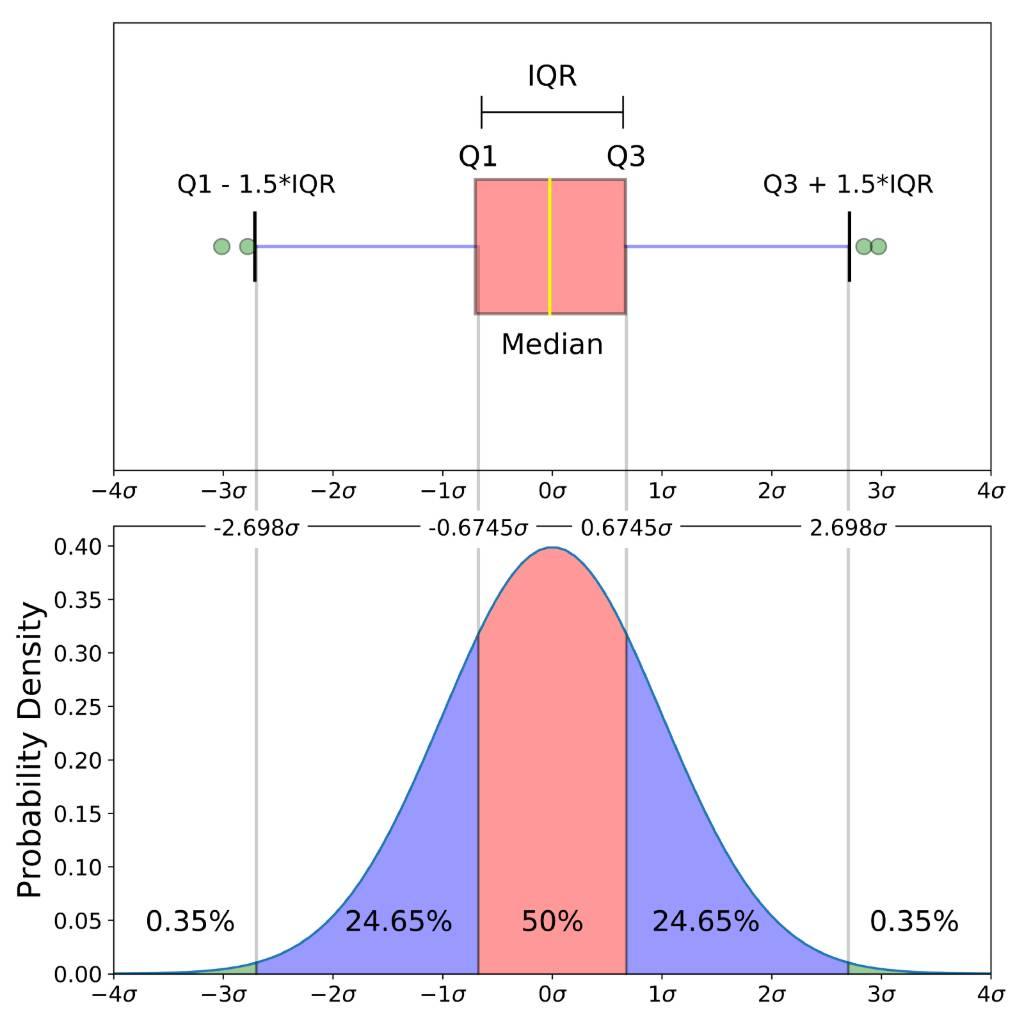The image above is a comparison of a boxplot of a nearly normal distribution and the probability density function (pdf) for a normal distribution. The reason why I am showing you this image is that looking at a statistical distribution is more commonplace than looking at a box plot. In other words, it might help you understand a boxplot.
This section will cover many things including:

• How outliers are (for a normal distribution) .7% of the data.
• What a “minimum” and a “maximum” are

## Probability Density Function

This part of the post is very similar to the 68–95–99.7 rule article, but adapted for a boxplot. To be able to understand where the percentages come from, it is important to know about the probability density function (PDF). A PDF is used to specify the probability of the random variable falling within a particular range of values, as opposed to taking on any one value. This probability is given by the integral of this variable’s PDF over that range - that is, it is given by the area under the density function but above the horizontal axis and between the lowest and greatest values of the range. This definition might not make much sense so let’s clear it up by graphing the probability density function for a normal distribution. The equation below is the probability density function for a normal distribution.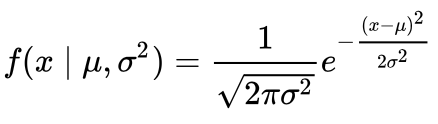Let’s simplify it by assuming we have a mean (μ) of 0 and a standard deviation (σ) of 1.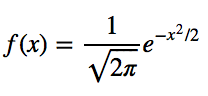This can be graphed using anything, but I choose to graph it using Python.

``````# Import all libraries for this portion of the blog post
import numpy as np
import matplotlib.pyplot as plt
%matplotlib inline
x = np.linspace(-4, 4, num = 100)
constant = 1.0 / np.sqrt(2*np.pi)
pdf_normal_distribution = constant * np.exp((-x**2) / 2.0)
fig, ax = plt.subplots(figsize=(10, 5));
ax.plot(x, pdf_normal_distribution);
ax.set_ylim(0);
ax.set_title('Normal Distribution', size = 20);
ax.set_ylabel('Probability Density', size = 20);
``````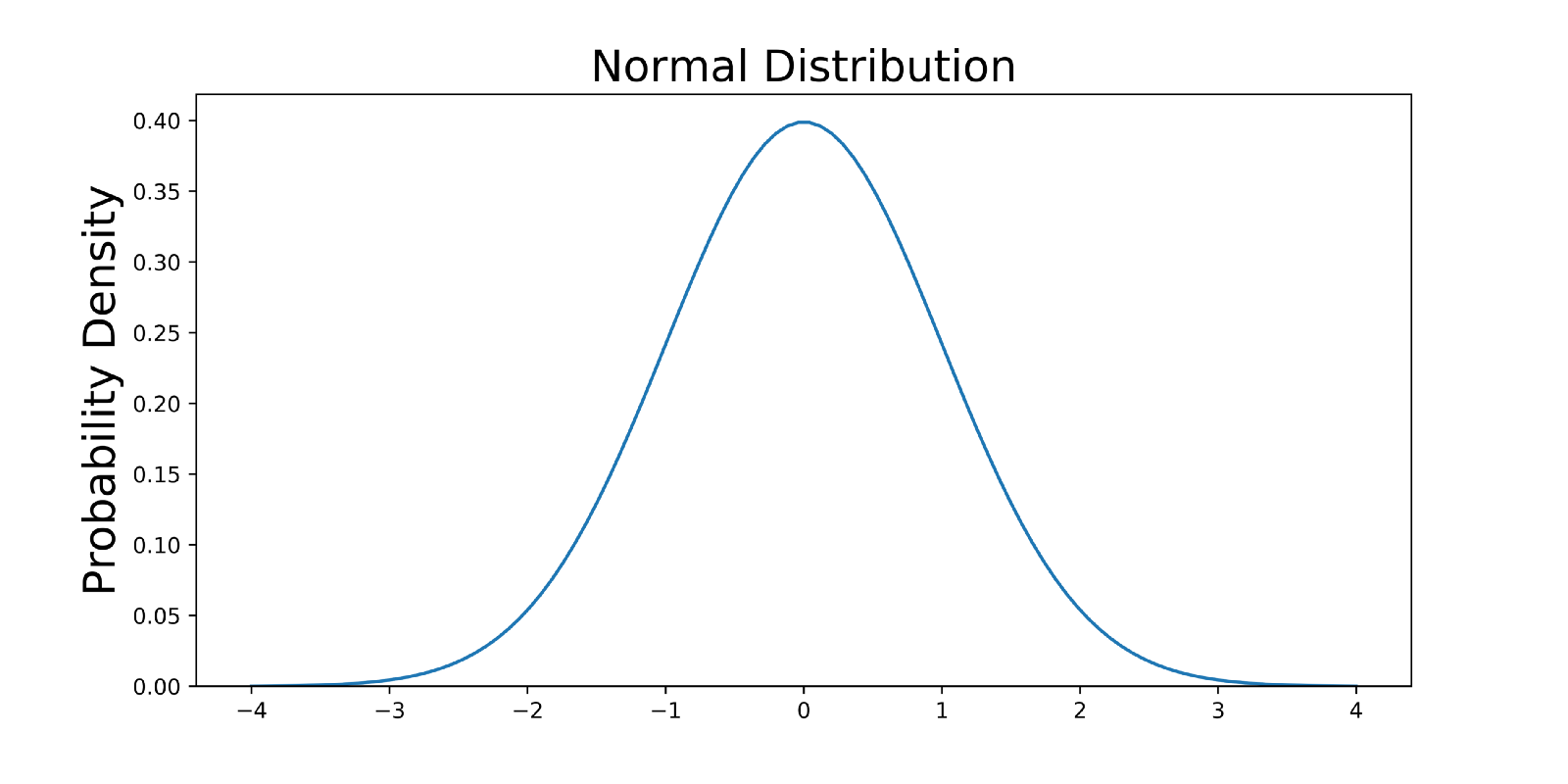The graph above does not show you the probability of events but their probability density. To get the probability of an event within a given range we will need to integrate. Suppose we are interested in finding the probability of a random data point landing within the interquartile range .6745 standard deviation of the mean, we need to integrate from -.6745 to .6745. This can be done with SciPy.

``````# Make PDF for the normal distribution a function
def normalProbabilityDensity(x):
constant = 1.0 / np.sqrt(2*np.pi)
return(constant * np.exp((-x**2) / 2.0) )
# Integrate PDF from -.6745 to .6745
result_50p, _ = quad(normalProbabilityDensity, -.6745, .6745, limit = 1000)
print(result_50p)
``````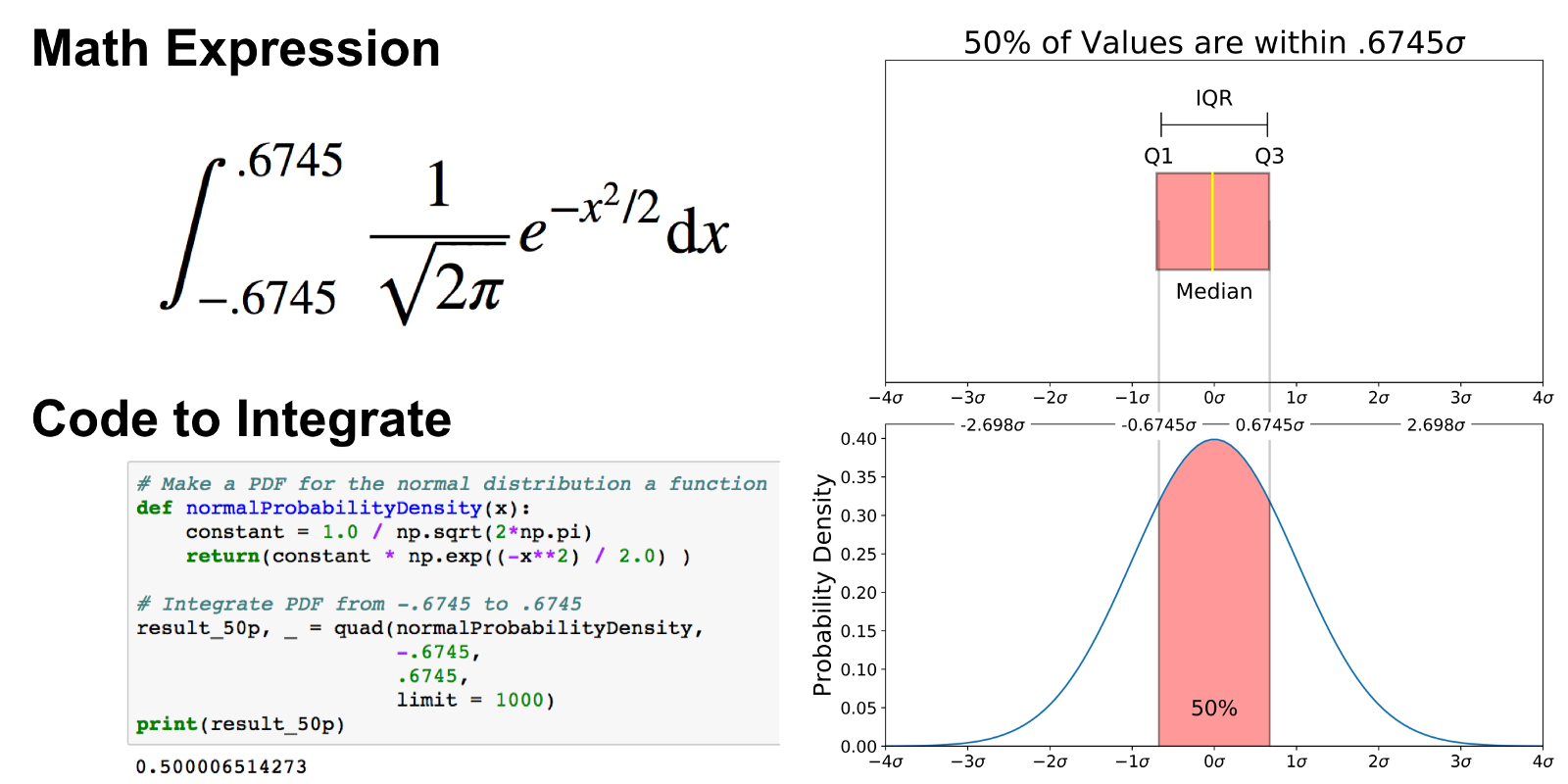The same can be done for “minimum” and “maximum”.

``````# Make a PDF for the normal distribution a function
def normalProbabilityDensity(x):
constant = 1.0 / np.sqrt(2*np.pi)
return(constant * np.exp((-x**2) / 2.0) )
# Integrate PDF from -2.698 to 2.698
-2.698,
2.698,
limit = 1000)
print(result_99_3p)
``````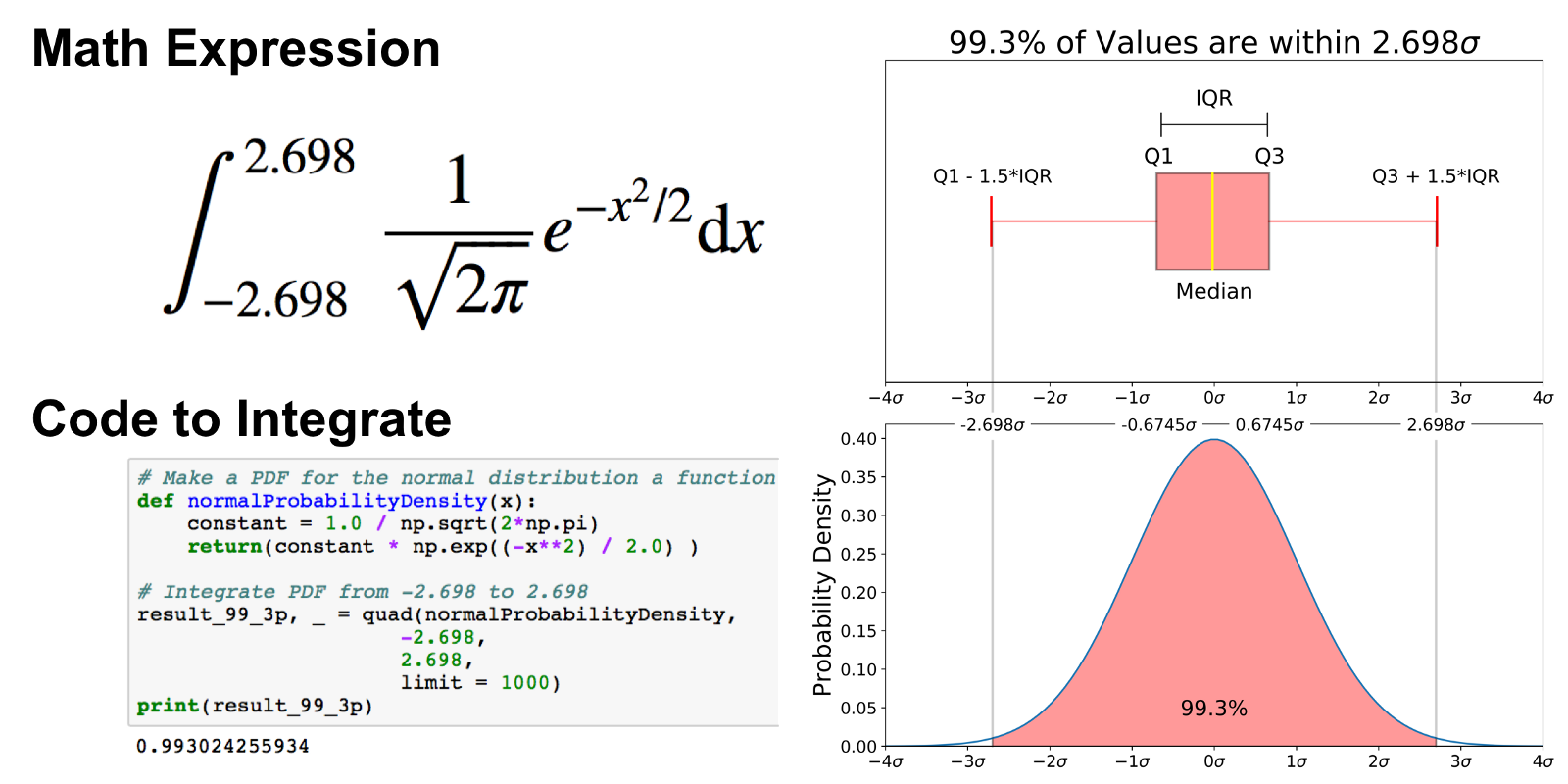As mentioned earlier, outliers are the remaining .7% percent of the data.
It is important to note that for any PDF, the area under the curve must be 1 (the probability of drawing any number from the function’s range is always 1).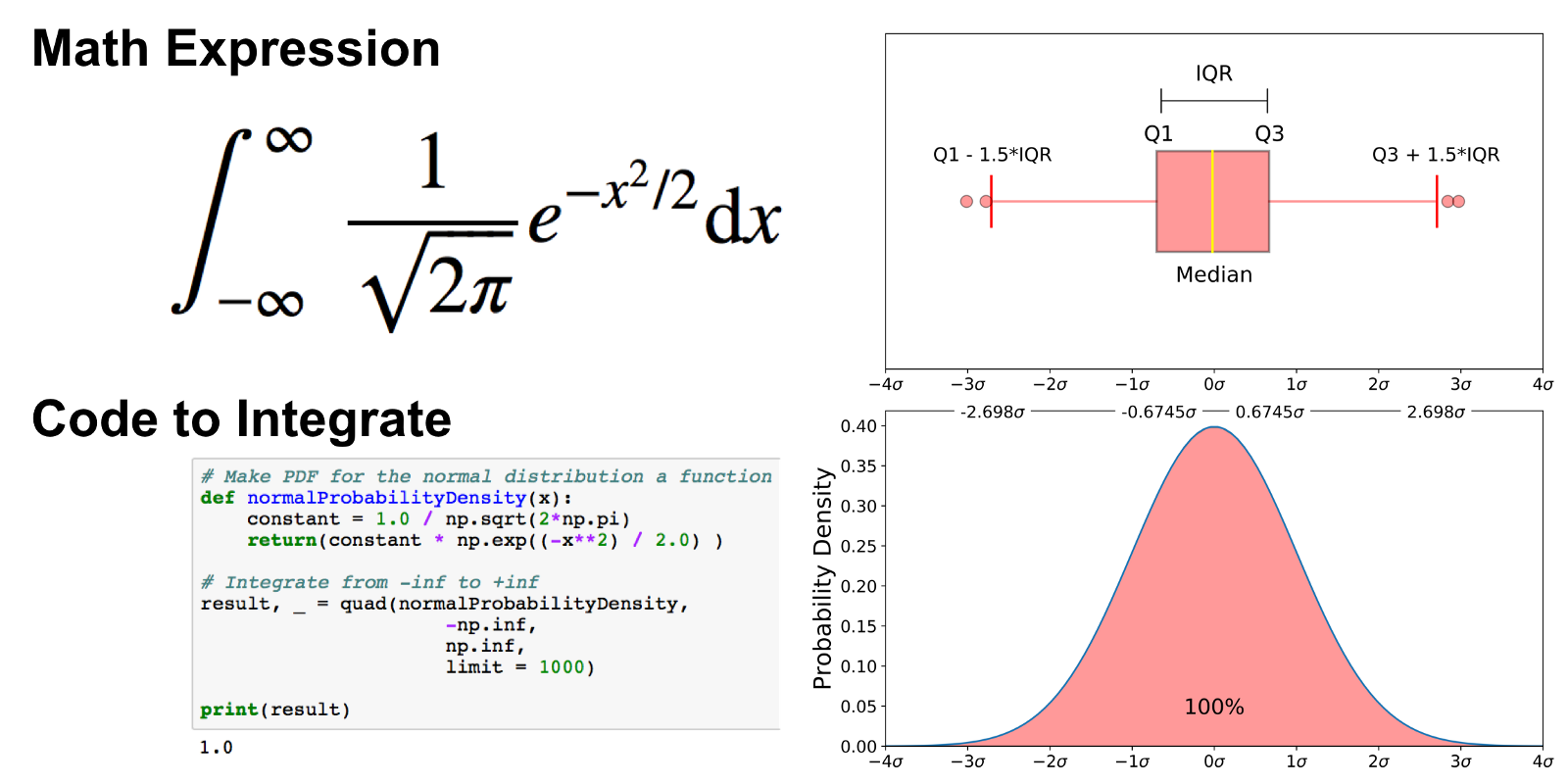Boxplots using Matplotlib, Pandas, and Seaborn Libraries (Python)

The video above is a free preview video from the Python for Data Visualization course.
In the last section, we went over a boxplot on a normal distribution, but as you obviously won’t always have an underlying normal distribution, let’s go over how to utilize a boxplot on a real dataset. To do this, we will utilize the Breast Cancer Wisconsin (Diagnostic) Dataset. If you don’t have a Kaggle account, you can download the dataset from my github.

The code below reads the data into a pandas dataframe.

``````import pandas as pd
import seaborn as sns
import matplotlib.pyplot as plt
# Put dataset on my github repo
``````

## Graph Boxplot

A boxplot is used below to analyze the relationship between a categorical feature (malignant or benign tumor) and a continuous feature (area_mean).
There are a couple ways to graph a boxplot through Python. You can graph a boxplot through seaborn, pandas, or seaborn.

### seaborn

The code below passes the pandas dataframe `df` into seaborn’s boxplot.

``````sns.boxplot(x='diagnosis', y='area_mean', data=df)
``````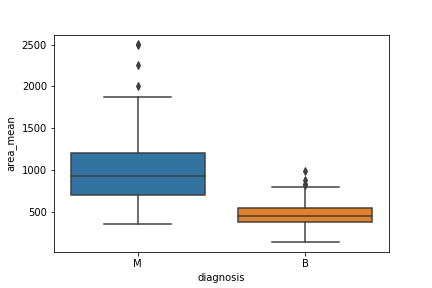### matplotlib

The boxplots you have seen in this post were made through matplotlib. This approach can be far more tedious, but can give you a greater level of control.

``````malignant = df[df['diagnosis']=='M']['area_mean']
benign = df[df['diagnosis']=='B']['area_mean']
fig = plt.figure()
ax.boxplot([malignant,benign], labels=['M', 'B'])
``````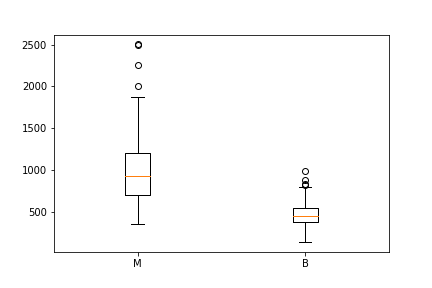Keep in mind that you can make this a lot prettier with a little bit of work.

### pandas

You can plot a boxplot by invoking `.boxplot()` on your DataFrame. The code below makes a boxplot of the area_mean column with respect to different diagnosis.

``````df.boxplot(column = 'area_mean', by = 'diagnosis');
plt.title('')
``````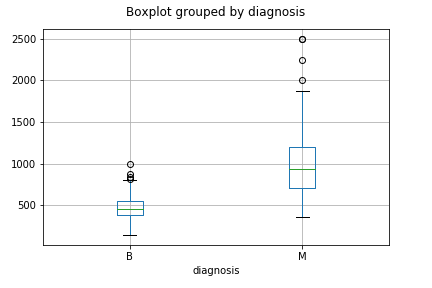## Notched Boxplot

The notched boxplot allows you to evaluate confidence intervals (by default 95% confidence interval) for the medians of each boxplot.

``````malignant = df[df['diagnosis']=='M']['area_mean']
benign = df[df['diagnosis']=='B']['area_mean']
fig = plt.figure()
ax.boxplot([malignant,benign], notch = True, labels=['M', 'B']);
``````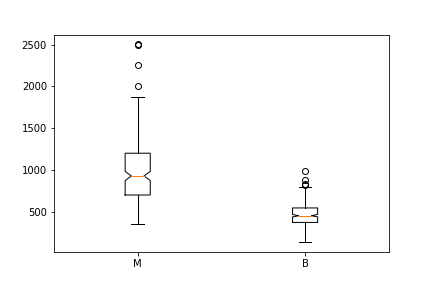## Interpreting a Boxplot

Data science is about communicating results so keep in mind you can always make your boxplots a bit prettier with a little bit of work (code here).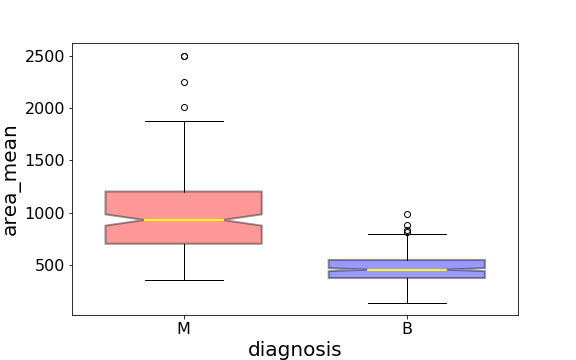Using the graph, we can compare the range and distribution of the area_mean for malignant and benign diagnosis. We observe that there is a greater variability for malignant tumor area_mean as well as larger outliers.
Also, since the notches in the boxplots do not overlap, you can conclude that with 95% confidence, that the true medians do differ.
Here are a few other things to keep in mind about boxplots:

1. Keep in mind that you can always pull out the data from the boxplot in case you want to know what the numerical values are for the different parts of a boxplot.
2. Matplotlib does not estimate a normal distribution first and calculates the quartiles from the estimated distribution parameters. The median and the quartiles are calculated directly from the data. In other words, your boxplot may look different depending on the distribution of your data and the size of the sample, e.g., asymmetric and with more or less outliers.

Hopefully this wasn’t too much information on boxplots. Future tutorials will take some this knowledge and go over how to apply it to understanding confidence intervals. My next tutorial goes over How to Use and Create a Z Table (standard normal table). If you any questions or thoughts on the tutorial, feel free to reach out in the comments below, through the YouTube video page, or through Twitter.